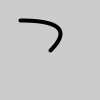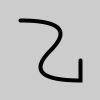Specifies vertex coordinates for quadratic Bezier curves.

## Examples#def setup():
py5.no_fill()
py5.stroke_weight(4)
py5.begin_shape()
py5.vertex(20, 20)
py5.end_shape()def setup():
py5.no_fill()
py5.stroke_weight(4)
py5.begin_shape()
py5.vertex(20, 20)
py5.vertex(80, 60)
py5.end_shape()


## Description#

Specifies vertex coordinates for quadratic Bezier curves. Each call to quadratic_vertex() defines the position of one control point and one anchor point of a Bezier curve, adding a new segment to a line or shape. The first time quadratic_vertex() is used within a begin_shape() call, it must be prefaced with a call to vertex() to set the first anchor point. This method must be used between begin_shape() and end_shape() and only when there is no MODE parameter specified to begin_shape(). Using the 3D version requires rendering with P3D.

## Signatures#

quadratic_vertex(
cx: float,  # the x-coordinate of the control point
cy: float,  # the y-coordinate of the control point
cz: float,  # the z-coordinate of the control point
x3: float,  # the x-coordinate of the anchor point
y3: float,  # the y-coordinate of the anchor point
z3: float,  # the z-coordinate of the anchor point
/,
) -> None